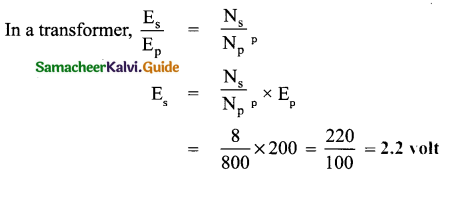Tamilnadu State Board New Syllabus Samacheer Kalvi 9th Science Guide Pdf Chapter 5 Magnetism and Electromagnetism Text Book Back Questions and Answers, Notes.

## Tamilnadu Samacheer Kalvi 9th Science Solutions Chapter 5 Magnetism and Electromagnetism

### 9th Science Guide Magnetism and Electromagnetism Text Book Back Questions and Answers

Question 1.
Which of the following converts electrical energy into mechanical energy?
(a) Motor
(b) Battery
(c) Generator
(d) Switch
(a) MotorQuestion 2.
Transformer works on ………………….
(a) AC only
(b DC only
(c) Both AC and DC
(a) AC only

Question 3.
The part of the AC generator that passes the current from the armature coil to theexternal circuit is .
(a) field magnet
(b) split rings
(c) slip rings
(d) brushes
d) brushesQuestion 4.
The unit of magnetic flux density is
(a) Weber
(b) weber/metre
(c) weber/metre2
(d) weber. metre2
(c) weber/metre2

II. Fill in the blanks :

1. The SI Unit of magnetic field induction is ……………..
Tesla

2. Devices which is used to convert high alternating current to low alternating current is ………………….
transformers3. An electric motor converts ………………
electrical energy into mechanical energy

4. A device for producing electric current is ……………
generator

III. Match the following :

 1. Magnetic material a) Oersted 2. Non-magnetic material b) Iron 3. Current and magnetism c) Induction 4. Electromagnetic induction d) Wood 5. Electric generator e) Faraday

1-b, 2-d, 3 -a, 4-e, 5-cIV. State whether true or false. If false, correct the statement

1. A generator converts mechanical energy into electrical energy.
True

2. Magnetic field lines always repel each other and do not intersect.
True

3. Fleming’s Left-hand rule is also known as the Dynamo rule.
False.
Correct statement: Fleming’s Left-hand rule is also known as the motor rule.4. The speed of rotation of an electric motor can be increased by decreasing the area of the coil.
False.
Correct statement: The speed of rotation of an electric motor can be increased by increasing the area of the coil.

5. A transformer can step up the direct current.
False.
Correct statement: A transformer can step up alternating current (AC).

6. In a step-down transformer the number of turns in the primary coil is greater than that of the number of turns in the secondary coil.
True.Question 1.
State Fleming’s Left Hand Rule.
It states that while stretching the three fingers of the left hand in a perpendicular manner with each other if the direction of the current is denoted by the middle finger of the left hand and the second finger is for the direction of the magnetic field then the thumb of the left hand denotes the direction of the force or movement of the conductor.

Question 2.
Define magnetic flux density.
The number of magnetic field lines crossing unit area kept normal to the direction of field lines is called magnetic flux density. Its unit is Wb/m2

Question 3.
List the main parts of an electric motor.
The main parts of an electric motor are:

1.  Shaft,
2. Primary and Secondary windings,
3. Ball bearings,
4. Armature,
5. Stator,
6. Commutator,
7. Brushes,
8. TerminalsQuestion 4.
Draw and label the diagram of an AC generator.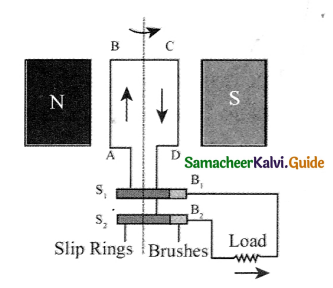PARTS
N, S – Permanent magnet
A B C D – Rectangular coil or armature.
S1,2 – Slip rings
B1, B2 – Carbon brushes

Question 5.
State the advantages of AC over DC.

• The Voltage of AC can be varied easily using a device called a transformer. The AC can be carried over long distances using step-up transformers. The loss of energy while distributing current in the form of AC is negligible.
• Direct current cannot be transmitted as such. The AC can be easily converted into DC. Generating AC is easier than DC. The AC can produce electromagnetic induction which is useful in several ways.Question 6.
Differentiate step up and step down transformer.

 Step-up transformer Step down transformer 1. It is a device used to raise the electric voltage (Vs > Vp) It is designed to reduce the voltage (Vs < Vp). 2. It has more turns on its secondary wind-ing in comparison to the primary one (Ns > Np). It has fewer turns on the secondary winding (Ns < Np). 3. It is used to start the electrical motor and step-up the windings. It is used to balance the ratio of voltage and current on the primary and secondary windings. 4. It takes a lot of voltage to initially start the motor turning. It increases the voltage but decreases the current. The secondary windings have fewer turns which mean low voltage and higher current while it is the opposite in the case of the primary winding. 5. It is found is a television and in power stations. It is found in laptops and phone chargers.

Question 7.
A portable radio has a built-in transformer so that it can work from the mains instead of batteries. Is this a step up or step down transformer? Give reason.
A step-down transformer is used in a portable radio in order to reduce the voltage.

Question 8.
State Faraday’s laws of electromagnetic induction.
First law: Whenever there is a change in magnetic flux linked with a coil, an electric current is induced. The induced potential difference lasts so long as there is a change in the magnetic flux linked with the coil.

Second law: The magnitude of the induced current is directly proportional to the rate of change of magnetic flux linked with the coil.

Question 1.
Explain the principle, construction, and working of a dc motor.
Principle: An electric motor works on the principle that a current-carrying conductor placed in a magnetic field experiences a force. The direction of force is given by Fleming’s left-hand rule.
Construction: An electric motor consists of the following main parts: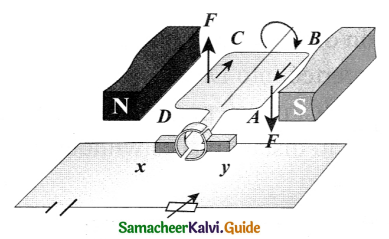Armature: It is a rectangular coil ABCD having a large number of turns of this insulated copper wire wound over a soft iron core. The armature is placed between the poles of the field magnet and it can be rotated about an axis perpendicular to the magnetic field lines.

Split rings (commutators): It consists of a cylindrical metal ring split into two halves S1 & S2 As the coil rotates, the split rings also rotate about the same axis of rotation. The function of the split ring is to reverse the direction of current in the coil after every half rotation.

Carbon brushes: Two graphite or flexible metal rods maintain a sliding contact with split rings S1and S2 alternately.

Battery: A battery of few cells is connected to the brushes. The current from the battery flows to the armature coil through the brushes and the split rings.

Working: A simple coil is placed inside two poles of a magnet. Now, look at the current-carrying conductor segment AB. The direction of the current is towards B, whereas in the conductor segment CD the direction is opposite. As the current is flowing in opposite directions in the segments AB and CD, the direction of the motion of the segments would be in opposite directions according to Fleming’s left-hand rule. When two ends of the coil experience force in opposite direction, they rotate.

If the current flow is along the line ABCD, then the coil will rotate in a clockwise direction first and then in an anticlockwise direction. If we want to make the coil rotate in any one direction, say clockwise, then the direction of the current should be along ABCD in the first half of the rotation and along DCBA in the second half of the rotation.

When the gap in the split ring commutator is aligned with terminals X and Y there is no flow of current in the coil. But, as the coil is moving, it continues to move forward bringing one of the split ring commutators in contact with the carbon brushes X and Y. The reversing of the current is repeated at each half rotation, giving rise to a continuous rotation of the coil.Question 2.
Explain two types of transformer.
Step-up transformer: The transformer used to change a low alternative voltage to a high alternating voltage is called a step-up transformer, ie (Vs > Vp)
In a step-up transformer, the number of turns in the secondary coil is more than the number of turns in the primary coil (Ns >Np)

Step down transformer: The transformer used to change a high alternating voltage to a low alternating voltage is called a step-down transformer (Vs< Vp). In a step-down transformer, the number of turns in the secondary coils is less than the number of turns in the primary coil (Ns < Np)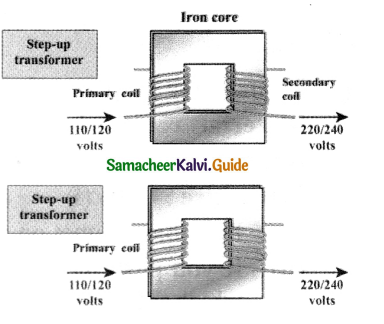Question 3.
Draw a neat diagram of an AC generator and explain its working.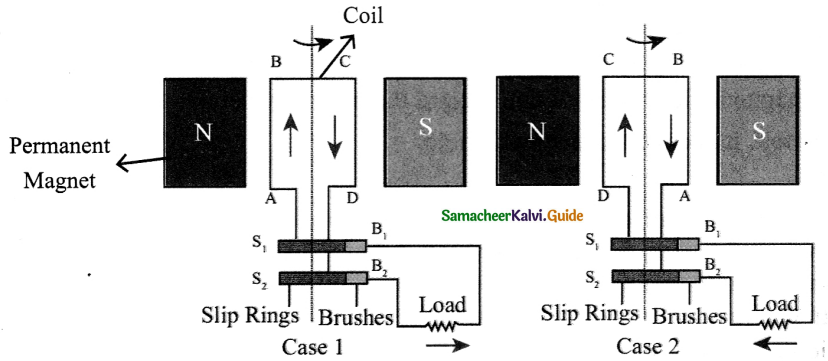• An alternating current (AC) generator, as shown in, consists of a rotating rectangular coil ABCD called armature placed between the two poles of a permanent magnet.
• The two ends of this coil are connected to two slip rings S1 and S2. The inner sides of these rings are insulated.
• Two conducting stationary brushes B1and B2 are kept separately on the rings S1and S2 respectively.
• The two rings S1 and S2 are internally attached to an axle.
• The axle may be mechanically rotated from outside to rotate the coil inside the magnetic field. Outer ends of the two brushes are connected to the external circuit.
• When the coil is rotated, the magnetic flux linked with the coil changes. This change in magnetic flux will lead to generation of induced current.
• The direction of the induced current, as given by Fleming’s Right Hand Rule, is along ABCD in the coil and in the outer circuit it flows from B2 to B1
• During the second half of rotation, the direction of current is along DCBA in the coil and in the outer circuit it flows from B1 to B2.
• As the rotation of the coif continues, the induced current in the external circuit is changing its direction for every half a rotation of the coil.

Intext Activities

ACTIVITY – 1

Put a magnet on a table and place some paper clips nearby. If you push the magnet slowly towards the paper clips, there will be a point at which the paper clips jump across and stick to the magnet. What do you understand from this?

Aim :
To study the property of a magnet.

Materials required :
Strong bar magnet, paper clips.

Procedure: Case (i)

• Put a bar magnet on a table and place some paper clips nearby.
• If you push the magnet slowly towards the paper clips, there will be a point at which the paper clips jump across and stick to the magnet. What do you understand from this?

Conclusion :

•  From the above activity, we can conclude that magnets have an invisible field all around them which attracts magnetic materials.
• In this space we can feel the force of attraction or repulsion due to the magnet.
• Thus magnetic field is the region around the magnet where its magnetic influence
can be felt. It is called a magnetic field.
[End of the activity]ACTIVITY – 2

Take a cardboard and thread a wire perpendicular through it. Connect the wire such that current flows up the wire. Switch on the circuit. Let the current flow. Place a magnetic compass on the cardboard and mark the position. Now move the magnet and mark the new position. If you join all the points you will find that it is a circle. Reverse the direction of the current, you will find the magnetic circles are clockwise.

Aim :
To observe the magnetic field around a straight conductor carrying current.

Materials required :
Thick copper wire (conductor), some connecting wires, battery, key, white cardboard, magnetic compass.

Procedure :

• Fix white cardboard horizontally.
• Make a small hole at its centre.
• Pass the thick copper wire through the hole, perpendicular to the cardboard.
• Join the ends of the thick copper wire to a battery and a key with the help of some connecting wires.
• Switch on the circuit. Let the current flow.
• Place a magnetic compass on the cardboard.
• Mark S and N point of the compass X and Y respectively on the cardboard.
• Move the compass such that S end touches Y.
• Now mark the N end as Z In the next step move the compass such that S end touch Z. Repeat the steps. .
• Now join all the points. What do you observe?
• Now keep the compass away from the centre and follow.

Observation:

• It is seen that it is a circle.
• We can draw another magnetic line and the magnetic lines are concentric circles and also we will find the magnetic lines are anti-clockwise.
• When the direction of current is reversed, the direction of magnetic lines of force
are clockwise.

[End of the activity]ACTIVITY – 3

You are given a long iron nail, insulation coated copper wire, and a battery. Can you make your own electromagnet?

Aim :

Materials required :
Iron nail insulated coated copped wire, battery, and paper clips.

Procedure :

• Take an iron nail, wind ten or more loops of insulated copper wire around it. However, make sure not to make the diameter of the loop more than a centimetre in width.
• Connect the ends of the wire to a key and a battery.
• Switch on the key and bring some paper clips close to the nail.
• Switch off the key. What do you observe?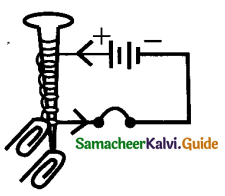Observation :
When the key is switched on, the electromagnet attracts the paper clips due to the current flowing through the coil wound around the iron nail. When the key is switched off, the paper clips detach from the iron nail

Conclusion:
Thus an electromagnet is a temporary magnet which behaves as a magnet in the presence of an electric current.### 9th Science Guide Magnetism and Electromagnetism Additional Important Questions and Answers

Question 1.
The direction of the magnetic field around a straight conductor carrying current can be determined by …………………
(a) Fleming’s left-hand rule
(b) Lenz’s law
(e) Right-hand thumb rule
(d) Fleming’s right-hand rule
(a) Fleming’s left-hand rule

Question 2.
The magnetic field produced due to a circular wire at its centre is _______.
(a) at 45° to the plane of the wire
(b) at 60° to the plane of the wire
(c) in the plane of the wire
(d) perpendicular to the plane of the wire
(d) perpendicular to the plane of the wireQuestion 3.
A magnetic field exerts no force on ………………
(a) stationary electric charge
(b) a magnet
(e) an electric charge moving perpendicular to its direction
(d) an unmagnetized iron bar.
(a) stationary electric charge

Question 4.
At the centre of a magnet, the magnetism is ______ .-
(a) zero
(b) same as the poles
(c) maximum
(d) minimum
(a) zeroQuestion 5.
Induced current flows through a coil ______.
(a) more than the period during which flux changes through it.
(b) less than the period during which flux changes through it
(c) only for the period during which flux changes through it
(d) None of the above
c) only for the period during which flux changes through it

Question 6.
Which of the following instruments works by electromagnet-ic induction?
(a) dynamo
(b) moving coil galvanometer
(d) simple motor
(a) dynamoQuestion 7.
For making a strong electromagnet the material of the core should be_______.
(a) brass
(b) laminated steel strips
(c) soft iron
(d) steel
(c) soft iron

Question 8.
Magnetic field lines determine ______
(a) the shape of the magnetic field
(b) only the direction of the magnetic field
(c) only the relative strength of the magnetic field
(d) both the direction and the relative strength of the magnetic field
(d) both the direction and the relative strength of the magnetic fieldQuestion 9.
A magnetic needle is kept in a non-uniform magnetic field. It experiences …………….
(a) a force and a torque
(b) a force but not a torque
(c) a torque but not a force
(d) neither a force nor a torque
(a) a force and a torque

Question 10.
The most suitable material for making permanent magnets is ………………..
(a) copper
(b) aluminum
(c) iron
(d) steel
(d) steelQuestion 11.
An electric generator converts
(a) electrical energy into mechanical energy
(b) mechanical energy into heat energy
(e) electrical energy into electrical energy
(d) mechanical energy into electrical energy
(d) mechanical energy into electrical energy

II. Fill in the blanks:

1. The magnetic field inside a _____ is uniform.

magnet

2. An AC generator is provided with …………… slip rings which rotate with the coil.
two3. The moving part of an electric motor is called ……………
shaft

4. A magnetic field is a ………………….quantity
Vector

5. The SI unit of magnetic field strength is ……………………
Tesla6. The laws of induction were given by ________

7. The relation between weber and Tesla is________
1wb = 1T × 1m2

8. Unlike magnetic poles ________whereas like poles ________
attract, repel

9. Magnetic lines of force never ______ each other.
intersect10. Sailors use ………………. to find direction in order to navigate on the sea.
Magnetic compass

11. The strongest natural magnet is ………………….
lodestone magnetite

12. The ______ produces its own magnetic field, which shields the earth’s ozone layer from the …………… and is important in navigation.
Earth, solar wind13. Sea turtles return to their birth beach many decades after they were born due to ……………..
geomagnetic imprinting

14. Magnetic field at a point is ………………. to the magnetic field lines.
tangential

15. ……………… is the number of magnetic field lines passing through a given area.
Magnetic flux16. The direction of the magnetic lines around a current-carrying conductor can be easily
understood using the ………….. rule.
right-hand thumb rule

17. The magnetic field lines are stronger near the ……………… and it diminishes as you go away from it.
current-carrying wire

18. A charge moving in a magnetic field, in a direction other than the direction of the magnetic field, experiences a force. It is called the ………………. force.
magnetic Lorentz19. When the conductor is perpendicular to the magnetic field, the force will be the …………….., When it is parallel to the magnetic field, the force will be ………………..
maximum, zero

20. An electric bell contains an …………………., consisting of coils of insulated wire wound around
iron rods.
electro magnet

21 . No force acts in a current carrying conductor when it is to the magnetic
field.III. Match the following :

Question 1.

 1. Electric generator a) Electro magnetic induction 2. Dynamo b) Fleming’s left hand rule 3. Magnetic field lines c) Slip rings 4. Electric motor d) North to south

1-c, 2 -a, 3-d, 4-b

Question 2.

 1. Electricity and magnetism a) Step-down transformer 2. Force on the conductor b) Hans Christian Oersted 3. Electric bell c) I L B 4. Ns

1-b, 2-c, 3-d, 4-aIV. State whether true or false. If false, correct the statement:

1. Fleming’s left-hand rule is also known as the dynamo rule.
False.
Correct statement: Fleming’s right-hand rule is also known as the dynamo rule.

2. Magnetic field lines always repel each other and do not intersect.
True.

3. A current flowing in a wire gives rise to a magnetic field.
True.4. The magnetic field lines due to a straight wire carrying current are parallel.
False.
Correct statement: The magnetic field lines due to a straight wire carrying current are concentric circles.

5. A generator converts mechanical energy into electrical energy.
True.

6. The magnetic field is a quantity that has magnitude only.
False
Correct statement: The magnetic field is a quantity that has magnitude and direction7. Magnetic fields do not interact with electric charges in motion.
False.
Correct statement: Magnetic fields do not interact with electric charges at rest.

8. The force on a charged particle is moving in a magnetic field is maximum when the angle between the direction of motion and field is 90°.
True.

9. An electric motor converts mechanical energy into electrical energy.
False.
Correct statement: An electric motor converts electrical energy into mechanical energy.10. The field at the centre of the long circular coil carrying current will be parallel straight lines.
True.

11. A wire with green insulation is usually the live wire of an electric supply.
False
Correct statement: A wire with green insulation is usually the earth wire of an electric supply.

12. An electric generator works on the principle of electromagnetic induction.
True.13. Permanent magnets are made of hard magnetic materials like alnico.
True.

14. The pole of a freely suspended magnet which points towards the north is called the south pole and what points towards south is celled north pole.
False.
Correct statement: The pole of a freely suspended magnet that points towards the north is called the north pole and that points towards the south are called a south pole.

15. The magnetic poles always exist in pairs.
True16. A galvanometer is an instrument that can detect the presence of a current is a circuit.
True

V. Assertion and Reason type questions :

Mark the correct choice as:
(a) If both assertion and reason are true and the reason is the correct explanation of assertion.
(b) If both assertion and reason are true but the reason is not the correct explanation of assertion.
(c) If the assertion is true but the reason is false.
(d) If the assertion is false but the reason is true

Question 1.
Assertion (A): When 2 long parallel wires, hanging freely are connected in parallel to a battery, they come closer to each other.
Reason (R): Wires carrying current in opposite direction repel each other.
(b) Both assertion and reason are true but the reason is not the correct explanation of the assertion

Reason: The wires are parallel to each other but the direction of current in it is in the same direction, so they attract each other. If the current in the wire is in opposite direction then wires repel each other.

Question 2.
Assertion (A) : The work done by a magnetic field on moving charge is zero.
Reason (R) : In a magnetic field force is perpendicular to the velocity.
(a) Both assertion and reason are true and the reason is the correct explanation ofQuestion 3.
Assertion (A): A current-carrying conductor experiences a force in a magnetic field.
Reason (R): The net charge on a current-carrying conductor is zero.
(b) Assertion and reason are true but the reason is not the correct explanation of the assertion

Reason: When a current-carrying conductor having no net charge is placed in a magnetic field, the free electrons of the conductor move towards the positive end of the conductor with the same drift velocity, hence magnetic force acts on them; The positive ions of the conductor being stationary, do not experience any magnetic field.

Question 4.
Assertion (A) : A magnetic field exerts a force on a moving charge in the same direction as the direction of the field itself.
Reason (R) : The direction of force is given by Fleming’s left-hand rule.
(d) Assertion is false but the reason is true
Reason: According to Fleming’s left-Hand Rule, the direction of the magnetic force on a moving charge is always perpendicular to the magnetic field.VI. Define the following :

Question 1.
Define the terms magnet and magnetism.
The substances which have the property of attracting small pieces of iron, nickel, cobalt, etc, are called magnets, and this property of attraction is called magnetism.

Question 2.
Define natural magnet.
It is a piece of lodestone, which is a black iron oxide (Fe3 O4) called magnetite. The word lodestone means a leading stone.Question 3.
Define artificial magnet.
Pieces of iron and other magnetic materials which can be made to acquire the properties of natural magnets are called artificial magnets.

Question 4.
Define magnetic field.
The region around a magnet within which its influence can be experienced is called a magnetic field.Question 5.
Define magnetic field lines of force.
The path in a magnetic field in which a unit north pole tends to move when allowed to do so is known as magnetic field lines of force.

Question 6.
Define magnetic flux.
Magnetic flux is the number of magnetic field lines passing through a given area. It is denoted by (Φ) and its unit is weber (Wb).Question 7.
Define magnetic shielding.
The process of stopping the magnetic field from entering into a region is called magnetic shielding.

Question 8.
Define magnetic effect of electric current.
A current-carrying conductor is always associated with a magnetic field around it is called the magnetic effect of current. It was first discovered by Hans Christian Oersted in 1820.

Question 9.
Define the right-hand thumb rule.
If the current-carrying conductor is held in the right hand such that the thumb points in the direction of the current, then the direction of the curl of the fingers will give the direction of the magnetic field.Question 10.
Define Fleming’s Left Hand Rule.
The law states that while stretching the three fingers of the left hand in a perpendicular manner with each other if the direction of the current is denoted by the middle finger of the left hand and the second finger is for the direction of the magnetic field then the thumb of the left hand denotes the direction of the force or movement of the conductor.

Question 11.
Define electromagnet.
lt is a solenoid with a soft iron core placed inside it. When current is passed through the solenoid, the soft iron core becomes a temporary magnet.

Question 12.
Define electric motor.
lt is a device to convert electrical energy into mechanical energy. It is based on the principle that when a current-carrying coil is placed in a magnetic field, it experiences a force.Question 13.
Define electromagnetic induction.
Whenever the magnetic lines of force passing through a closed-circuit change, a voltage and hence a current is induced in it. This phenomenon is called electromagnetic r induction. It was discovered by Michael Faraday in 1831.

Question 14.
Define Fleming’s Right Hand Rule.
Stretch the thumb, forefinger, and middle finger of your right hand mutually perpendicular to each other. If the fore finger indicates the direction of the magnetic field and the thumb indicates the direction of motion of the conductor, then the middle finger will indicate the direction of the induced current. Fleming’s Right-hand rule is also called the “generator rule”.Question 15.
Define an electric generator or a dynamo.
lt is a device to convert mechanical energy into electrical energy. It is based on the principle of electromagnetic induction that a current is induced in a closed circuit when the magnetic field passing through it changes.

Question 16.
Define a Transformer.
The transformer is a device used for converting low voltage into high voltage and high voltage into low voltage. It works on the principle of electromagnetic induction.Question 17.
Define step up and step down transformer.
Step-up transformer: The transformer used to change a low alternating voltage to a high alternating voltage is called a step-up transformer, (ie) (Vs > Vp).
Step down transformer: The transformer used to change a high alternating voltage to a low alternating voltage is called a step-down transformer (Vs < Vp).

Question 1.
Mention the properties of magnetic lines of force.
Magnetic lines of force are closed continuous curves, extending through the body of the magnet.
Magnetic lines of force start from the North Pole and end at the South Pole.

Question 2.
Give the uses of magnets in everyday life.

•  Used in radio and stereo speakers
• Used in almirah and refrigerator doors to snap them closed.
• In medicine, magnetic resonance imaging (MRI) scanners expose the inner parts of the patient’s body for detailed examination by doctors.Question 3.
No, if two magnetic lines of force intersect then there will be two tangents and hence two directions of the magnetic field at the point of intersection. This is not possible.

Question 4.
Why does a compass needle get deflected when brought near a bar magnet?
The magnetic field of the magnet exerts a force on both the poles of the compass needle. The forces experienced by the two poles are equal and opposite. These two forces form a couple which deflects the compass needle.Question 5.
Draw magnetic field lines around a bar magnet.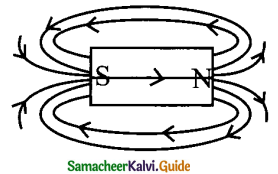Question 6.
Give some uses of electromagnets.

• It is used in factories and cranes to lift heavy iron ingots and steel, scraps from one place to another.
• Used in hospitals to remove iron splinters from the eyes of the patients.
• Used in electric bells, relays, electric switches etc.

Question 7.
Two coils A and B of insulated wire are kept close to each other. Coil A is connected to a galvanometer. While coil B is connected to a battery through a key. What would happen if.
(i) a current is passed through coil B by plugging the key?
(ii) the current is stopped by removing the plug from the key?
(i) If a current is passed through coil B by plugging the key, the needle of the galvanometer instantly jumps to one side and just as quickly returns to zero, indicating a momentary current in coil – A (As the current in coil B changes, the magnetic field associated with it also changes).

(ii) If the current is stopped by removing the plug from the key :
The needle of the galvanometer in coil – A momentarily moves but to the opposite side. It means that now the current flows in the opposite direction in coil – A.Question 1.
Explain the principle, construction, and working of an AC generator.

• An alternating current (AC) generator, consists of a rotating rectangular coil ABCD called armature placed between the two poles of a permanent magnet.
• The two ends of this coil are connected to the two slip rings S1 and S2. The inner sides of these rings are insulated.
• Two conducting stationary brushes B1 and B2 are kept separately on the rings S1 and S2 respectively. The two rings S1 and S2 are internally attached to an axle.
• The axle may be mechanically rotated from outside to rotate the coil inside the magnetic field. Outer ends of the two bushes are connected to the external circuit.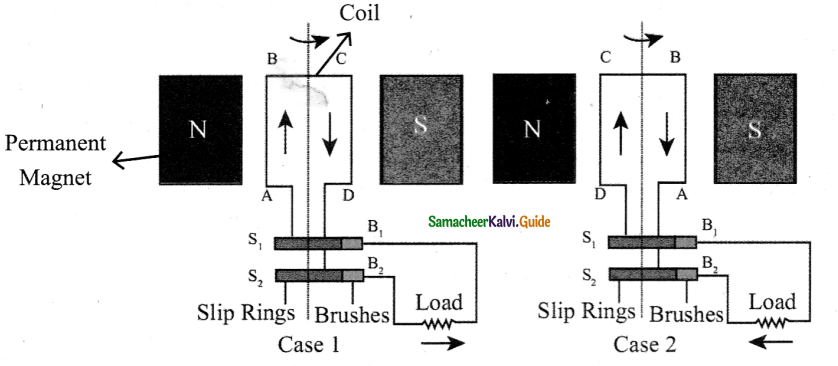When the coil is rotated, the magnetic flux linked with the coil changes. This change in magnetic flux will lead to generation of induced current.
• The direction of the induced current, as given by Fleming’s Right Hand Rule, is along ABCD in the coil and in the outer circuit it flows from
B2 to B1
• During the second half of rotation, the direction of current is along DCBA in the coil and in the outer circuit it flows from B1 , to B2
• As the rotation of the coil continues, the induced current in the external circuit is changing its direction for every half a rotation of the coil.Question 2.
Explain the working of following devices.
(i) Speaker
(ii) Magnetic levitation trains.
(i) Inside the speaker, the electromagnet is placed in front of a permanent magnet. The permanent magnet is fixed firmly in position whereas the electromagnet is mobile. As pulses of electricity pass through the coil of the electromagnet, the direction of its magnetic field is rapidly changed. This means that it is, in turn, attracted to and repelled from the permanent magnet vibrating back and forth. The electromagnet is attached to a cone made of a flexible material such as paper or plastic which amplifies these vibrations, pumping sound waves into the surrounding air towards our ears.

(ii) Magnetic levitation (Maglev) is a method by which an object is suspended with no support other than magnetic fields. In Maglev trains two sets of magnets are used, one set to repel and push the train up off the track,’ then another set to move the floating train ahead at great speed without friction. In this technology, there is no moving part. The train travels along a guide way of magnets which controls the train’s stability and speed using the basic principles of magnets.

Question 3.
Explain the application of electromagnets in the field of medical system.
Nowadays electromagnetic fields play a key role in advanced medical equipment such as hyperthermia treatments for cancer, implants and magnetic resonance imaging (MRI). In a, sophisticated equipments working based on electromagnetism can scan minute details of the human body.

Many of the medical equipments such as scanners, X-ray equipments and other equipments also use principle of electromagnetism for their functioningQuestion 4.
Explain the properties of magnetic lines of force.

• Magnetic lines of force are closed, continuous curves, extending through the body of the magnet.
• Magnetic lines of force start from the North Pole and end at the South Pole.
• Magnetic lines of force never intersect.
• They will be maximum at the poles than at the equator.
• The tangent drawn at any point on the curved line gives the direction of the magnetic field.

IX. Numerical Problems:

Question 1.
A 0.4 m wire, stretched horizontally, carries an electric current of 15 A, in a magnetic field whose magnetic field intensity is 0.1 N/Am. What is the magnitude on the wire?
Given: l = 0.4 m, I = 15A
Magnetic induction B = 0.1 N/Am
Solution:
Force to be used F = B I l
= 0.1 × 15 × 0.4
F = 0.6N

Question 2.
A wire 10 cm long carrying a current of 1.5 A is held in a uniform magneÑic field in which B = 10-3 T. Calculate the force on the wire if it is held perpendicular to the lines of the magnetic field.
Given : Length of the wire l = 10 cm = 0.10 m
Current I= 1.5 A
Magnetic induction B = 10-3T
Solution:
ForceF = B I l
= 10-3 × 1.5 × 0.10
= 1.5 × 10-3 N.Question 3.
A conductor of length 50 cm carrying a current of 5 A is placed perpendicular to a magnetic field of induction 2×10 -3T. Find the force on the conductor.
Solution :
Force on the conductor = ILB
= 5 × 50 × 10-2 × 2 × 10-3
= 5 × 10-3

Question 4.
A current-carrying conductor of a certain length, kept perpendicular to the magnetic field experiences a force F. What will be the force if the current is increased four times, the length is halved and the magnetic field is tripled?
Solution :
F = I L B = (4I) × $$\left(\frac{L}{2}\right)$$ × (3 B) =6 F
Therefore, the force increases six times.

Question 5.
The primary coil of a transformer has 800 turns and the secondary coil has 8 turns. It is connected to a 220 V ac supply. What will be the output voltage?
Solution: